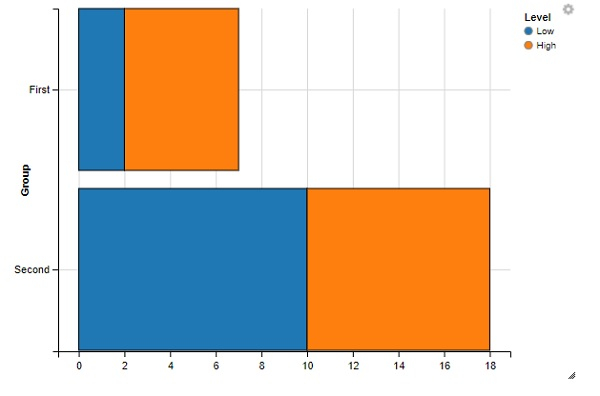# How to create horizontal stacked bar chart using ggvis in R?

R ProgrammingServer Side ProgrammingProgramming

To create stacked bar chart using ggvis, we can follow the below steps −

• First of all, create a data frame.
• Create the horizontal stacked bar chart with layer_rects function of ggvis package.

## Create the data frame

Let's create a data frame as shown below −

Live Demo

Group<-c("First","Second","First","Second")
Level<-c("Low","Low","High","High")
Count<-c(2,10,5,8)
df<-data.frame(Group,Level,Count)
df

On executing, the above script generates the below output(this output will vary on your system due to randomization) −

  Group Level Count
1 First  Low   2
2 Second Low   10
3 First  High  5
4 Second High  8

## Create the horizontal stacked bar chart

Loading ggvis package and creating horizontal stacked bar chart with layer_rects function −

library(ggvis)
Group<-c("First","Second","First","Second")
Level<-c("Low","Low","High","High")
Count<-c(2,10,5,8)
df<-data.frame(Group,Level,Count)
df %>% ggvis(y = ~Group, fill = ~Level) %>% compute_stack(stack_var = ~Count,
group_var = ~Group) %>% layer_rects(x = ~stack_lwr_, x2 = ~stack_upr_, height =
band())

### Output